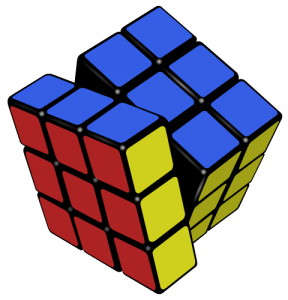# Algebraic Groups

by | Jan 12, 2021 | Math Learning

# Introduction to Algebraic Groups

One of the most fundamental algebraic structures in mathematics is the group.

A group is a set of elements paired with an operation that satisfies the following four conditions:

I. It is closed under an operation (represented here by “+”, although it does not necessarily mean addition):
For all elements a and b in the set S, a+b is also in S.

II. It contains an identity element (often written as “e”):
There is some element e in the set S such that for every element a in S, a+e = e+a = a.

III. The operation is associative:
For all a, b, and c in the set S, (a+b)+c = a+(b+c).

IV. Inverses exist:
For every element a in the set S, there is an a-1 in S such that a+a-1 = a-1+a = e We will look at some examples of groups and sets that aren’t groups.

Example 1. Integers under addition, (ℤ, +).
I. The group operation is addition. For any two integers n and m, n+m is also an integer.
II. The identity element is 0 because for any integer n, n+0 = 0+n = n.
IV. Inverses exist. The inverse of any integer n is -n, and n + (-n) = (-n) + n = 0. Therefore, (ℤ, +) is a group.

Example 2. Integers under multiplication, (ℤ, ⋅)
I. The group is closed under multiplication, because for any two integers n and m, n⋅m is also an integer.
II. The identity element is 1. For any integer n, n⋅1 = 1⋅n = n.
III. Multiplication is associative.
IV. Inverses do NOT exist. For any integer n, 1/n is not an integer except when n=1. Since the the set does not meet all four criteria, (ℤ, ⋅) is not a group.

Example 3. The set of rational numbers not including zero, under multiplication (ℚ – 0, )
I. The group is closed under multiplication.
II. 1 is the identity element.
III. Multiplication is associative.
IV. Inverses exist in this group.

If q is a rational number, 1/q is also a rational number. And q⋅ (1/q) = (1/q) ⋅ q = 1.

Notice that if 0 were in this set, it would not be a group because 0 has no inverse. Therefore, ℚ – 0 is a group.

Example 4. The set {0, 1, 2} under addition
I. This set is not closed under addition because 1 + 2 = 3, and 3 is not part of the set. Therefore, the set cannot be a group.

Example 5. The trivial group {e} This group consists only of the identity element. We don’t need to specify the operation here because it works for both multiplication and addition.

For addition, e=0. 0+0=0 so the group is closed under addition, has an identity element, and is closed under inverses (and addition is associative).

For multiplication, e=1 and similarly, 1⋅1 = 1.

The examples here involved only numbers, but there are many different types of groups.

For example, the ways to transform a triangle is called a Dihedral Group, with the group operation being the act of rotating or reflecting the shape around an axis. The possible permutations of a Rubik’s Cube are also a group, with the operation being a sequence of moves. It’s powerful to know that a set is a group because it gives you an understanding of how the elements will always behave. Groups have many other properties that are useful to mathematicians, and there is a whole field of study built off of this knowledge called Group Theory.The set of permutations of a Rubik’s Cube is considered an algebraic group.

Featured Bundles